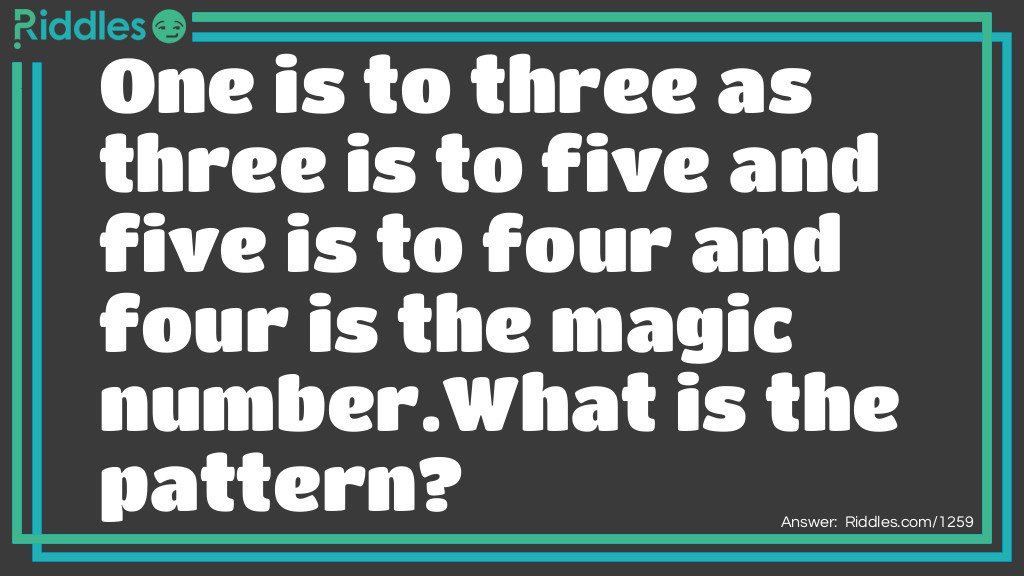Riddle: One is to three as three is to five and five is to four and four is the magic number.  What is the pattern?
Answer: One has three letters in the word three has five letters in it five has four letters and four has four letters in it (if you try more numbers they will always come back to the number four: so four is the magic number)One is to three as three is to five riddle Riddle Meme with riddle and answer.
Riddle: What did the triangle say to the circle?
Riddle: If a boy blows 18 bubbles, Then pops 6 eats 7 and then He pops 5 and blows 1. How many are left?
Riddle: The ages of a father and son add up to 66. The father's age is the son's age reversed. How old could they be? (3 possible solutions).
Answer: 51 and 15. 42 and 24. 60 and 06.
Riddle: What two whole, positive numbers that have a one-digit answer when multiplied and a two-digit answer when added?